# pedmod: Pedigree Models

The pedmod package provides functions to estimate models for pedigree data. Particularly, the package provides functions to estimate mixed models of the form:

\begin{align*} Y_{ij} \mid \epsilon_{ij} = e &\sim \text{Bin}(\Phi(\vec\beta^\top\vec x_{ij} + e), 1) \\ \vec\epsilon_i = (\epsilon_{i1}, \dots, \epsilon_{in_i})^\top &\sim N^{(n_i)}\left(\vec 0, \sum_{l = 1}^K\sigma_l^2 C_{il} \right) \end{align*}

where $$Y_{ij}$$ is the binary outcome of interest for individual $$j$$ in family/cluster $$i$$, $$\vec x_{ij}$$ is the individual’s known covariates, $$\Phi$$ is the standard normal distribution’s CDF, and $$\text{Bin}$$ implies a binomial distribution such if $$z\sim \text{Bin}(p, n)$$ then the density of $$z$$ is:

$f(z) = \begin{pmatrix} n \\ z \end{pmatrix}p^z(1 - p)^{n-z}$

A different and equivalent way of writing the model is as:

\begin{align*} Y_{ij} \mid \epsilon_{ij} = e &= \begin{cases} 1 & \vec\beta^\top\vec x_{ij} + e > 0 \\ 0 & \text{otherwise} \end{cases} \\ \vec\epsilon_i = (\epsilon_{i1}, \dots, \epsilon_{in_i})^\top &\sim N^{(n_i)}\left(\vec 0, I_{n_i} + \sum_{l = 1}^K\sigma_l^2 C_{il} \right) \end{align*}

where $$I_{n_i}$$ is the $$n_i$$ dimensional identity matrix which comes from the unshared/individual specific random effect. This effect is always included. The models are commonly known as liability threshold models or mixed probit models.

The $$C_{il}$$s are known scale/correlation matrices where each of the $$l$$’th types correspond to a type of effect. An arbitrary number of such matrices can be passed to include e.g. a genetic effect, a maternal effect, a paternal, an effect of a shared adult environment etc. Usually, these matrices are correlation matrices as this simplifies later interpretation and we will assume that all the matrices are correlation matrices. A typical example is that $$C_{il}$$ is two times the kinship matrix in which case we call:

$\frac{\sigma_l^2}{1 + \sum_{k = 1}^K\sigma_k^2}$

the heritability. That is, the proportion of the variance attributable to the the $$l$$’th effect which in this case is the direct genetic effect. The scale parameters, the $$\sigma_k^2$$s, may be the primary interest in an analysis. The scale in the model cannot be identified. That is, an equivalent model is:

\begin{align*} Y_{ij} \mid \epsilon_{ij} = e &= \begin{cases} 1 & \sqrt\phi\vec\beta^\top\vec x_{ij} + e > 0 \\ 0 & \text{otherwise} \end{cases} \\ \vec\epsilon_i = (\epsilon_{i1}, \dots, \epsilon_{in_i})^\top &\sim N^{(n_i)}\left(\vec 0, \phi\left(I_{n_i} + \sum_{l = 1}^K\sigma_l^2 C_{il}\right) \right) \end{align*}

for any $$\phi > 0$$. A common option other than $$\phi = 1$$ is to set $$\phi = (1 + \sum_{l = 1}^K \sigma_l^2)^{-1}$$. This has the effect that

$\frac{\sigma_l^2}{1 + \sum_{k = 1}^K\sigma_k^2} = \phi\sigma_l^2$

is the proportion of variance attributable to the $$l$$’th effect (assuming all $$C_{il}$$ matrices are correlation matrices). Moreover, $$\phi$$ is the proportion of variance attributable to the individual specific effect.

The parameterizations used in the package are $$\phi = 1$$ which we call the direct parameterizations and $$(1 + \sum_{l = 1}^K \sigma_l^2)^{-1}$$ which we call the standardized parameterizations. The latter have the advantage that it is easier to interpret as the scale parameters are the proportion of variance attributable to each effect (assuming that only correlation matrices are used) and the $$\sqrt\phi\vec\beta$$ are often very close the estimate from a GLM (that is, the model without the other random effects) when the covariates are unrelated to random effects that are added to the model. The latter makes it easy to find starting values.

For the above reason, two parameterization are used. For the direct parameterization where $$\phi = 1$$, we work directly with $$\vec\beta$$, and we use $$\theta_l = \log\sigma_l^2$$. For the standardized parameterization where $$\phi = (1 + \sum_{l = 1}^K \sigma_l^2)^{-1}$$, we work with $$\phi = (1 + \sum_{l = 1}^K \sigma_l^2)^{-1}$$, $$\vec\gamma = \sqrt\phi\vec\beta$$, and

$\phi\sigma_l^2 = \frac{\exp(\psi_l)}{1 +\sum_{l = 1}^k\exp(\psi_l)}\Leftrightarrow\sigma_l^2 = \exp(\psi_l).$

This package provides randomized quasi-Monte Carlo methods to approximate the log marginal likelihood for these types of models with an arbitrary number scale matrices, $$K$$, and the derivatives with respect to $$(\vec\beta^\top, 2\log\sigma_1,\dots, 2\log\sigma_K)^\top$$ (that is, we work with $$\psi_k = 2\log\sigma_k$$) or $$(\vec\gamma^\top, \psi_1, \dots, \psi_K)$$.

In some cases, it may be hypothesized that some individuals are less effected by e.g. their genes than others. A model to incorporate such effects is implemented in the pedigree_ll_terms_loadings function. See the Individual Specific Loadings section for details and examples.

We have re-written the Fortran code by Genz and Bretz (2002) in C++, made it easy to extend from a log marginal likelihood approximation to other approximations such as the derivatives, and added less precise but faster approximations of the $$\Phi$$ and $$\Phi^{-1}$$. Our own experience suggests that using the latter has a small effect on the precision of the result but can yield substantial reduction in computation times for moderate sized families/clusters.

The approximation by Genz and Bretz (2002) have already been used to estimate these types of models (Pawitan et al. 2004). However, not having the gradients may slow down estimation substantially. Moreover, our implementation supports computation in parallel which is a major advantage given the availability of multi-core processors.

Since the implementation is easy to extend, possible extensions are:

1. Survival times using mixed generalized survival models (Liu, Pawitan, and Clements 2017) with a similar random effect structure as the model shown above. This way, one avoids dichotomizing outcomes and can account for censoring.
2. Generalized linear mixed model with binary, binomial, ordinal, or multinomial outcomes with a probit link. The method we use here may be beneficial if the number of random effects per cluster is not much smaller then the number observations in each cluster. This is used for imputation in the mdgc package.

## Installation

The package can be installed from GitHub by calling:

remotes::install_github("boennecd/pedmod", build_vignettes = TRUE)

The package can also be installed from CRAN by calling:

install.packages("pedmod")

The code benefits from being build with automatic vectorization so having e.g.  -O3 in the CXX14FLAGS flags in your Makevars file may be useful.

## Example

We start with a simple example only with a direct genetic effect. We have one type of family which consists of two couples which are related through one of the parents being siblings. The family is shown below.

# create the family we will use
fam <- data.frame(id = 1:10, sex = rep(1:2, 5L),
father = c(NA, NA, 1L, NA, 1L, NA, 3L, 3L, 5L, 5L),
mother = c(NA, NA, 2L, NA, 2L, NA, 4L, 4L, 6L, 6L))

# plot the pedigree
library(kinship2)
ped <- with(fam, pedigree(id = id, dadid = father, momid = mother, sex = sex))
plot(ped)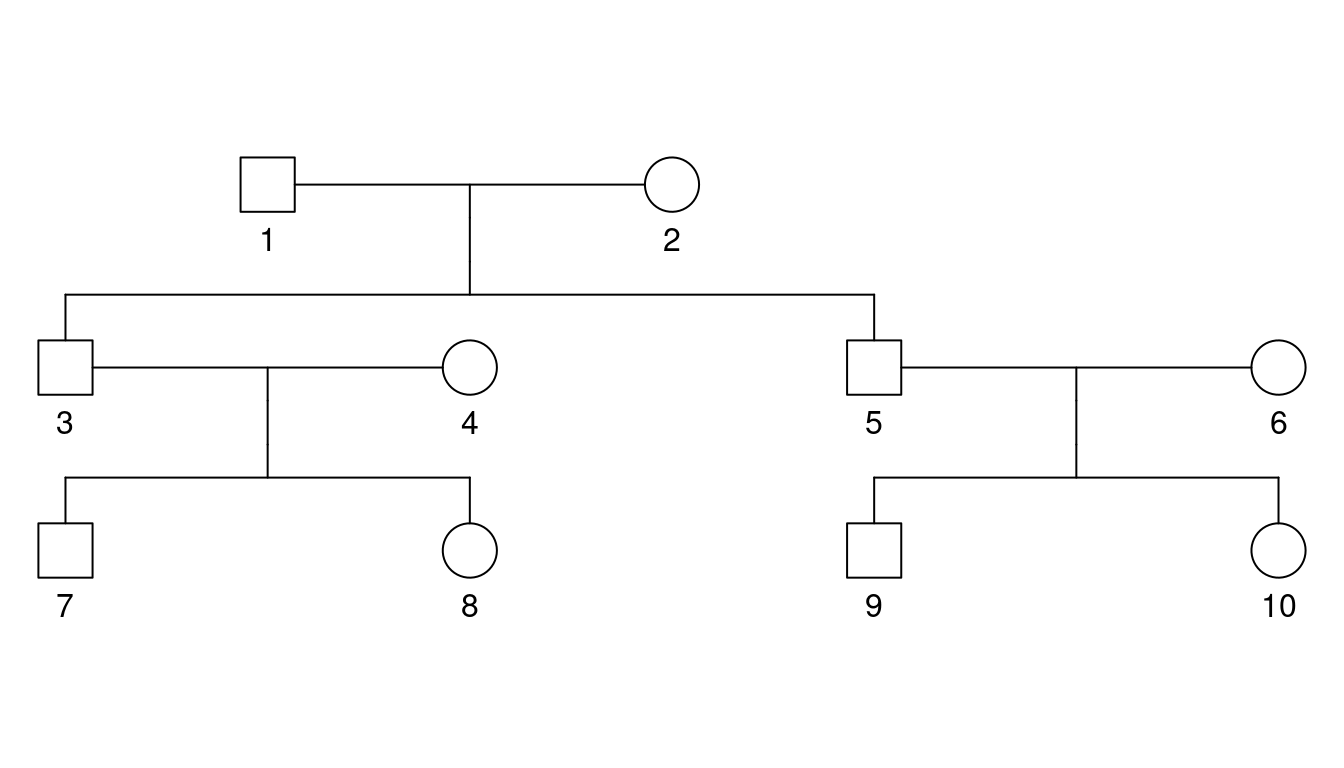We set the scale matrix to be two times the kinship matrix to model the direct genetic effect. Each individual also has a standard normally distributed covariate and a binary covariate. Thus, we can simulate a data set with a function like:

# simulates a data set.
#
# Args:
#   n_fams: number of families.
#   beta: the fixed effect coefficients.
#   sig_sq: the scale parameter.
sim_dat <- function(n_fams, beta = c(-3, 1, 2), sig_sq = 3){
# setup before the simulations
Cmat <- 2 * kinship(ped)
n_obs <- NROW(fam)
Sig <- diag(n_obs) + sig_sq * Cmat
Sig_chol <- chol(Sig)

# simulate the data
out <- replicate(
n_fams, {
# simulate covariates
X <- cbind((Intercept) = 1, Continuous = rnorm(n_obs),
Binary = runif(n_obs) > .5)

# assign the linear predictor + noise
eta <- drop(X %*% beta) + drop(rnorm(n_obs) %*% Sig_chol)

# return the list in the format needed for the package
list(y = as.numeric(eta > 0), X = X, scale_mats = list(Cmat))
}, simplify = FALSE)

# add attributes with the true values and return
attributes(out) <- list(beta = beta, sig_sq = sig_sq)
out
}

The model is

\begin{align*} Y_{ij} &= \begin{cases} 1 & \beta_0 + \beta_1 X_{ij} + \beta_2 B_{ij} + G_{ij} + R_{ij} > 0 \\ 0 & \text{otherwise} \end{cases} \\ X_{ij} &\sim N(0, 1) \\ B_{ij} &\sim \text{Bin}(0.5, 1) \\ (G_{i1}, \dots, G_{in_{i}})^\top &\sim N^{(n_i)}(\vec 0, \sigma^2 C_{i1}) \\ R_{ij} &\sim N(0, 1)\end{align*}

where $$C_{i1}$$ is two times the kinship matrix and $$X_{ij}$$ and $$B_{ij}$$ are observed covariates. We can now estimate the model with a simulated data set as follows:

# simulate a data set
set.seed(27107390)
dat <- sim_dat(n_fams = 400L)

library(pedmod)
ll_terms <- pedigree_ll_terms(dat, max_threads = 4L)
system.time(start <- pedmod_start(ptr = ll_terms, data = dat, n_threads = 4L))
#>    user  system elapsed
#>  15.623   0.020   3.945

# log likelihood without the random effects and at the starting values
start$logLik_no_rng #>  -1690 start$logLik_est # this is unreliably/imprecise
#>  -1619

# estimate the model
system.time(
opt_out <- pedmod_opt(
ptr = ll_terms, par = start$par, abs_eps = 0, use_aprx = TRUE, n_threads = 4L, maxvls = 25000L, rel_eps = 1e-3, minvls = 5000L)) #> user system elapsed #> 45.853 0.003 11.554 The results of the estimation are shown below: # parameter estimates versus the truth rbind(opt_out = head(opt_out$par, -1),
opt_out_quick = head(start  $par, -1), truth = attr(dat, "beta")) #> (Intercept) Continuous Binary #> opt_out -2.872 0.9689 1.878 #> opt_out_quick -2.844 0.9860 1.857 #> truth -3.000 1.0000 2.000 c(opt_out = exp(tail(opt_out$par, 1)),
opt_out_quick = exp(tail(start  $par, 1)), truth = attr(dat, "sig_sq")) #> opt_out opt_out_quick truth #> 2.908 2.812 3.000 # log marginal likelihoods print(start$logLik_est, digits = 8) # this is unreliably/imprecise
#>  -1618.5064
print(-opt_out$value , digits = 8) #>  -1618.4045 We emphasize that we set the rel_eps parameter to 1e-3 above which perhaps is fine for this size of a data set but may not be fine for larger data sets for the following reason. Suppose that we have $$i = 1,\dots,m$$ families/clusters and suppose that we estimate the log likelihood term for each family with a variance of $$\zeta$$. This implies that the variance of the log likelihood for all the families is $$\zeta m$$. Thus, the precision we require for each family’s log likelihood term needs to be proportional to $$\mathcal O(m^{-1/2})$$ if we want a fixed number of precise digits for the log likelihood for all number of families. The latter is important e.g. for the profile likelihood curve we compute later and also for the line search used by some optimization methods. Thus, one may need to reduce rel_eps and increase maxvls when there are many families. ### Minimax Tilting The minimax tilting method suggested by Botev (2017) is also implemented. The method is more numerically stable when the marginal likelihood terms are small (for instance with large clusters) or for certain problems. However, there is some overhead in the implementation of the method as underflow becomes an issue. This requires more care which increases the computation time. We estimate the model below with the minimax tilting using the use_tilting argument. # perform the optimization. We start with finding the starting values set.seed(60941821) system.time( start_tilt <- pedmod_start(ptr = ll_terms, data = dat, n_threads = 4L, use_tilting = TRUE)) #> user system elapsed #> 19.812 0.000 4.982 # estimate the model system.time( opt_out_tilt <- pedmod_opt( ptr = ll_terms, par = start_tilt$par, abs_eps = 0, use_aprx = TRUE,
n_threads = 4L, use_tilting = TRUE,
maxvls = 25000L, rel_eps = 1e-3, minvls = 5000L))
#>    user  system elapsed
#> 120.978   0.012  30.381

The results of the estimation are shown below:

# parameter estimates versus the truth
rbind(opt_out_tilt  = head(opt_out_tilt$par, -1), opt_out = head(opt_out$par     , -1),
truth         = attr(dat, "beta"))
#>              (Intercept) Continuous Binary
#> opt_out_tilt      -2.874     0.9694  1.879
#> opt_out           -2.872     0.9689  1.878
#> truth             -3.000     1.0000  2.000
c(opt_out_tilt  = exp(tail(opt_out_tilt$par, 1)), opt_out = exp(tail(opt_out$par, 1)),
truth         = attr(dat, "sig_sq"))
#> opt_out_tilt      opt_out        truth
#>        2.912        2.908        3.000

# log marginal likelihoods
print(start        $logLik_est, digits = 8) # this is unreliably/imprecise #>  -1618.5064 print(start_tilt$logLik_est, digits = 8) # this is unreliably/imprecise
#>  -1618.5602

print(-opt_out     $value , digits = 8) #>  -1618.4045 print(-opt_out_tilt$value     , digits = 8)
#>  -1618.4068

### Alternative Parameterization

As an alternative to the direct parameterization we use above, we can also use the standardized parameterization. Below are some illustrations which you may skip.

#####
# transform the parameters and check that we get the same likelihood
std_par <- direct_to_standardized(opt_out$par, n_scales = 1L) std_par # the standardized parameterization #> (Intercept) Continuous Binary #> -1.4528 0.4901 0.9502 1.0673 opt_out$par # the direct parameterization
#> (Intercept)  Continuous      Binary
#>     -2.8718      0.9689      1.8783      1.0673

# we can map back as follows
par_back <- standardized_to_direct(std_par, n_scales = 1L)
all.equal(opt_out$par, par_back, check.attributes = FALSE) #>  TRUE # the proportion of variance of each effect attr(par_back, "variance proportions") #> Residual #> 0.2559 0.7441 # the proportion match exp(tail(opt_out$par, 1)) / (exp(tail(opt_out$par, 1)) + 1) #> #> 0.7441 # compute the likelihood with either parameterization set.seed(1L) eval_pedigree_ll(ptr = ll_terms, par = opt_out$par, maxvls = 10000L,
minvls = 1000L, rel_eps = 1e-3, use_aprx = TRUE, abs_eps = 0)
#>  -1618
#> attr(,"n_fails")
#>  10
#> attr(,"std")
#>  0.004053
set.seed(1L)
eval_pedigree_ll(ptr = ll_terms, par = std_par    , maxvls = 10000L,
minvls = 1000L, rel_eps = 1e-3, use_aprx = TRUE, abs_eps = 0,
standardized = TRUE)
#>  -1618
#> attr(,"n_fails")
#>  10
#> attr(,"std")
#>  0.004053

# we can also get the same gradient with an application of the chain rule
jac <- attr(
standardized_to_direct(std_par, n_scales = 1L, jacobian = TRUE),
"jacobian")

set.seed(1L)
g1 <- eval_pedigree_grad(ptr = ll_terms, par = opt_out$par, maxvls = 10000L, minvls = 1000L, rel_eps = 1e-3, use_aprx = TRUE, abs_eps = 0) set.seed(1L) g2 <- eval_pedigree_grad(ptr = ll_terms, par = std_par, maxvls = 10000L, minvls = 1000L, rel_eps = 1e-3, use_aprx = TRUE, abs_eps = 0, standardized = TRUE) all.equal(drop(g1 %*% jac), g2, check.attributes = FALSE) #>  TRUE The model can also be estimated with the standardized parameterization: # perform the optimization. We start with finding the starting values system.time(start_std <- pedmod_start( ptr = ll_terms, data = dat, n_threads = 4L, standardized = TRUE)) #> user system elapsed #> 5.688 0.000 1.431 # the starting values are close standardized_to_direct(start_std$par, n_scales = 1L)
#> (Intercept)  Continuous      Binary
#>     -2.8435      0.9858      1.8566      1.0332
#> attr(,"variance proportions")
#> Residual
#>   0.2625   0.7375
start$par #> (Intercept) Continuous Binary #> -2.844 0.986 1.857 1.034 # this may have required different number of gradient and function evaluations start_std$opt$counts #> function gradient #> 31 31 start$opt$counts #> function gradient #> 48 48 # estimate the model system.time( opt_out_std <- pedmod_opt( ptr = ll_terms, par = start_std$par, abs_eps = 0, use_aprx = TRUE,
n_threads = 4L, standardized = TRUE,
maxvls = 25000L, rel_eps = 1e-3, minvls = 5000L))
#>    user  system elapsed
#>  32.580   0.000   8.227

# we get the same
standardized_to_direct(opt_out_std$par, n_scales = 1L) #> (Intercept) Continuous Binary #> -2.8708 0.9691 1.8772 1.0674 #> attr(,"variance proportions") #> Residual #> 0.2559 0.7441 opt_out$par
#> (Intercept)  Continuous      Binary
#>     -2.8718      0.9689      1.8783      1.0673

# this may have required different number of gradient and function evaluations
opt_out_std$counts #> function gradient #> 15 10 opt_out$counts
#>       37       12

### Stochastic Quasi-Newton Method

The package includes a stochastic quasi-Newton method which can be used to estimate the model. This may be useful for larger data sets or in situations where pedmod_opt “get stuck” near a maximum. The reason for the latter is presumably that pedmod_opt (by default) uses the BFGS method which does not assume any noise in the gradient or the function. We give an example below of how to use the stochastic quasi-Newton method provided through the pedmod_sqn function.

# fit the model with the stochastic quasi-Newton method
set.seed(46712994)
system.time(
sqn_out <- pedmod_sqn(
ptr = ll_terms, par = start$par, abs_eps = 0, use_aprx = TRUE, n_threads = 4L, rel_eps = 1e-3, step_factor = .1, maxvls = 25000L, minvls = 1000L, n_it = 400L, n_grad_steps = 10L, n_grad = 100L, n_hess = 400L)) #> user system elapsed #> 342.704 0.008 85.981 # show the log marginal likelihood ll_wrapper <- function(x) eval_pedigree_ll( ptr = ll_terms, x, maxvls = 50000L, minvls = 1000L, abs_eps = 0, rel_eps = 1e-4, n_threads = 4L) print(ll_wrapper(sqn_out$par), digits = 8)
#>  -1618.4635
#> attr(,"n_fails")
#>  151
#> attr(,"std")
#>  0.00073468344
print(ll_wrapper(opt_out$par), digits = 8) #>  -1618.4063 #> attr(,"n_fails") #>  169 #> attr(,"std") #>  0.00073978509 # compare the parameters rbind(optim = opt_out$par,
sqn   = sqn_out$par) #> (Intercept) Continuous Binary #> optim -2.872 0.9689 1.878 1.067 #> sqn -2.841 0.9734 1.865 1.039 # plot the marginal log likelihood versus the iteration number lls <- apply(sqn_out$omegas, 2L, ll_wrapper)
par(mar = c(5, 5, 1, 1))
plot(lls, ylab = "Log marginal likelihood", bty = "l", pch = 16,
lines(smooth.spline(seq_along(lls), lls))
grid()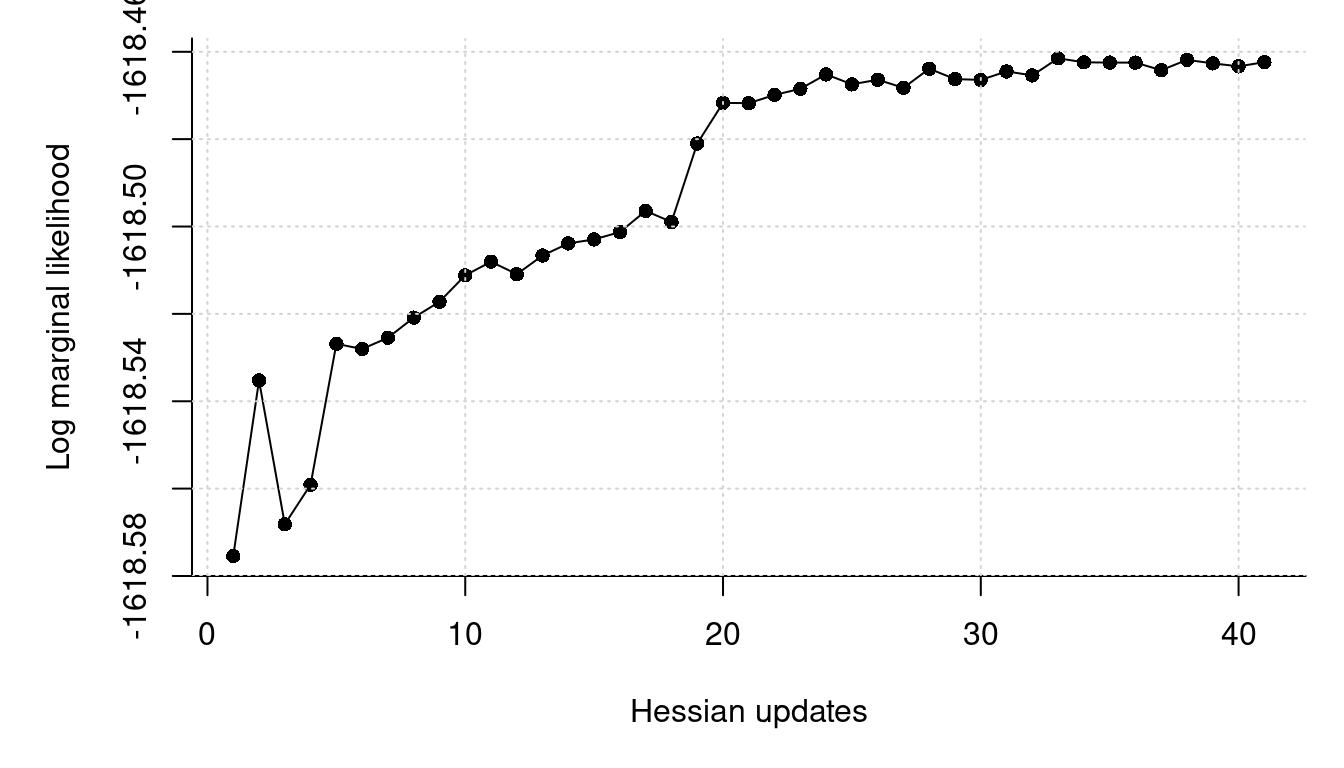# perhaps we could have used fewer samples in each iteration
set.seed(46712994)
system.time(
sqn_out_few <- pedmod_sqn(
ptr = ll_terms, par = start$par, abs_eps = 0, use_aprx = TRUE, n_threads = 4L, rel_eps = 1e-3, step_factor = .1, maxvls = 25000L, minvls = 1000L, n_grad_steps = 20L, # we take more iterations n_it = 2000L, # but use fewer samples in each iteration n_grad = 20L, n_hess = 100L)) #> user system elapsed #> 339.594 0.008 84.987 # compute the marginal log likelihood and compare the parameter estimates print(ll_wrapper(sqn_out_few$par), digits = 8)
#>  -1618.4489
#> attr(,"n_fails")
#>  156
#> attr(,"std")
#>  0.00074678963

rbind(optim       = opt_out    $par, sqn = sqn_out$par,
sqn (few) = sqn_out_few$par) #> (Intercept) Continuous Binary #> optim -2.872 0.9689 1.878 1.067 #> sqn -2.841 0.9734 1.865 1.039 #> sqn (few) -2.845 0.9533 1.877 1.035 ### Profile Likelihood Curve We can compute a profile likelihood curve like this: # assign the scale parameter at which we will evaluate the profile likelihood rg <- range(exp(tail(opt_out$par, 1) / 2) * c(.5, 2),
sqrt(attr(dat, "sig_sq")) * c(.9, 1.1))
sigs <- seq(rg, rg, length.out = 10)
sigs <- sort(c(sigs, exp(tail(opt_out$par, 1) / 2))) # compute the profile likelihood ll_terms <- pedigree_ll_terms(dat, max_threads = 4L) pl_curve_res <- lapply(sigs, function(sig){ # set the parameters to pass beta <- start$beta_no_rng
sig_sq_log <- 2 * log(sig)
beta_scaled <- beta * sqrt(1 + sig^2)

# optimize like before but using the fix argument
opt_out_quick <- pedmod_opt(
ptr = ll_terms, par = c(beta_scaled, sig_sq_log), maxvls = 1000L,
abs_eps = 0, rel_eps = 1e-2, minvls = 100L, use_aprx = TRUE, n_threads = 4L,
fix = length(beta) + 1L)
opt_out <- pedmod_opt(
ptr = ll_terms, par = c(opt_out_quick$par, sig_sq_log), abs_eps = 0, use_aprx = TRUE, n_threads = 4L, fix = length(beta) + 1L, # we changed these parameters maxvls = 25000L, rel_eps = 1e-3, minvls = 5000L) # report to console and return message(sprintf("\nLog likelihood %.5f (%.5f). Estimated parameters:", -opt_out$value, -opt_out_quick$value)) message(paste0(capture.output(print( c(opt_out$par, Scale = sig))), collapse = "\n"))

list(opt_out_quick = opt_out_quick, opt_out = opt_out)
})

We can construct an approximate 95% confidence interval using an estimated cubic smoothing spline for the profile likelihood (more sigs points may be needed to get a good estimate of the smoothing spline):

# get the critical values
alpha <- .05
crit_val <- qchisq(1 - alpha, 1)

# fit the cubic smoothing spline
pls <- -sapply(pl_curve_res, function(x) x$opt_out$value)
smooth_est <- smooth.spline(sigs, pls)

# check that we have values within the bounds
max_ml <- -opt_out$value ll_diffs <- 2 * (max_ml - pls) stopifnot(any(head(ll_diffs, length(ll_diffs) / 2) > crit_val), any(tail(ll_diffs, length(ll_diffs) / 2) > crit_val)) # find the values max_par <- tail(opt_out$par, 1)
lb <- uniroot(function(x) 2 * (max_ml - predict(smooth_est, x)$y) - crit_val, c(min(sigs) , exp(max_par / 2)))$root
ub <- uniroot(function(x) 2 * (max_ml - predict(smooth_est, x)$y) - crit_val, c(exp(max_par / 2), max(sigs)))$root

# the confidence interval
c(lb, ub)
#>  1.259 2.528
c(lb, ub)^2 # on the variance scale
#>  1.586 6.392

A caveat is that issues with the $$\chi^2$$ approximation may arise on the boundary of the scale parameter ($$\sigma = 0$$; e.g.  see https://stats.stackexchange.com/a/4894/81865). Notice that the above may fail if the estimated profile likelihood is not smooth e.g. because of convergence issues. We can plot the profile likelihood and highlight the critical value as follows:

par(mar = c(5, 5, 1, 1))
plot(sigs, pls, bty = "l",
pch = 16, xlab = expression(sigma), ylab = "Profile likelihood")
grid()
lines(predict(smooth_est, seq(min(sigs), max(sigs), length.out = 100)))
abline(v = exp(tail(opt_out$par, 1) / 2), lty = 2) # the estimate abline(v = sqrt(attr(dat, "sig_sq")), lty = 3) # the true value abline(v = lb, lty = 3) # mark the lower bound abline(v = ub, lty = 3) # mark the upper bound abline(h = max_ml - crit_val / 2, lty = 3) # mark the critical value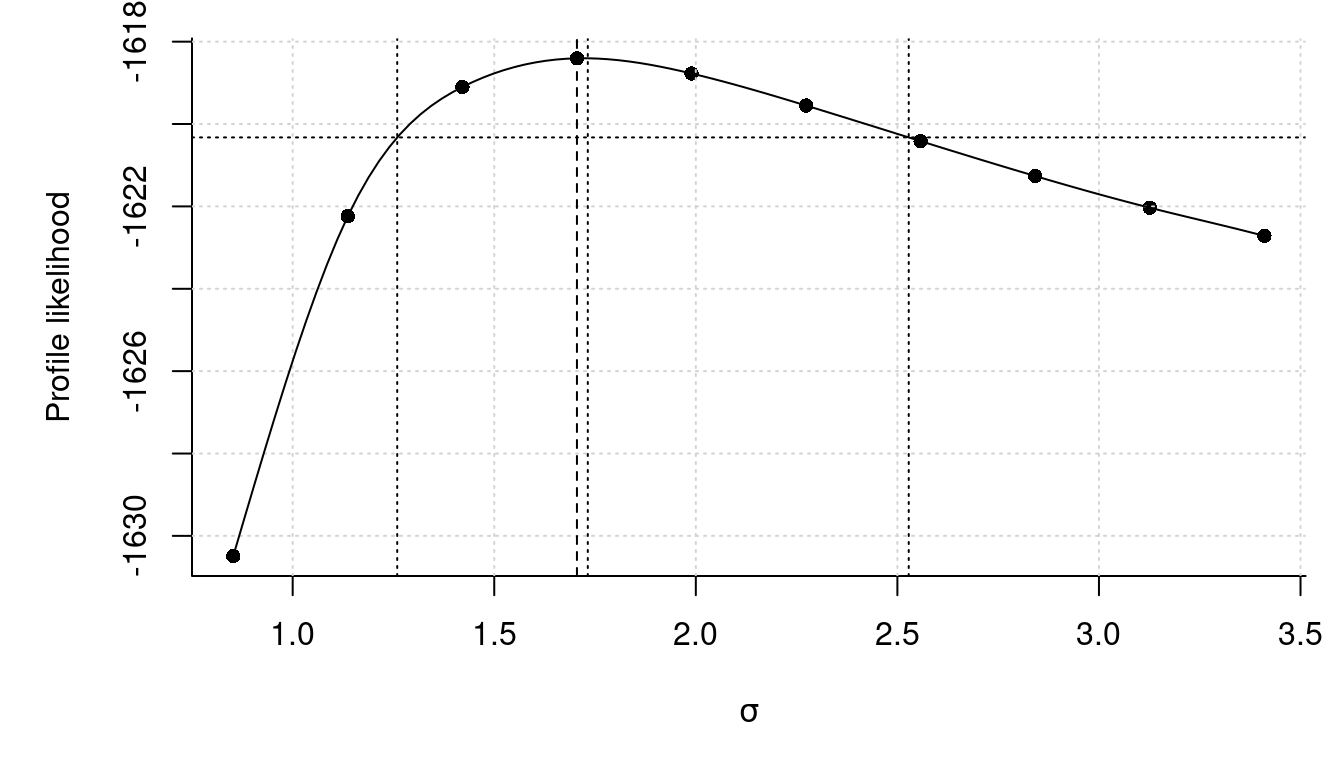The pedmod_profile function is a convenience function to do like above. An example of using the pedmod_profile function is provided below: # find the profile likelihood based confidence interval prof_res <- pedmod_profile( ptr = ll_terms, par = opt_out$par, delta = .5, maxvls = 10000L,
minvls = 1000L, alpha = .05, abs_eps = 0, rel_eps = 1e-4, which_prof = 4L,
use_aprx = TRUE, n_threads = 4L, verbose = TRUE)
#> The estimate of the standard error of the log likelihood is 0.00264089. Preferably this should be below 0.001
#>
#> Finding the lower limit of the profile likelihood curve
#> LogLike: -1619.7619 at         0.567300
#> LogLike: -1619.7602 at         0.567300
#> LogLike: -1624.4396 at         0.067300
#> LogLike: -1624.4340 at         0.067300
#> LogLike: -1620.8786 at         0.405931. Lb, target, ub: -1620.8786, -1620.3315, -1619.7602
#> LogLike: -1620.8729 at         0.405931. Lb, target, ub: -1620.8729, -1620.3315, -1619.7602
#> LogLike: -1620.3400 at         0.477023. Lb, target, ub: -1620.3400, -1620.3315, -1619.7602
#> LogLike: -1620.3378 at         0.477023. Lb, target, ub: -1620.3378, -1620.3315, -1619.7602
#>
#> Finding the upper limit of the profile likelihood curve
#> LogLike: -1619.3169 at         1.567300
#> LogLike: -1619.3037 at         1.567300
#> LogLike: -1621.2055 at         2.067300
#> LogLike: -1621.1781 at         2.067300
#> LogLike: -1620.2903 at         1.838320. Lb, target, ub: -1621.1781, -1620.3315, -1620.2903
#> LogLike: -1620.2683 at         1.838320. Lb, target, ub: -1621.1781, -1620.3315, -1620.2683
#> LogLike: -1620.4497 at         1.878615. Lb, target, ub: -1620.4497, -1620.3315, -1620.2683
#> LogLike: -1620.4236 at         1.878615. Lb, target, ub: -1620.4236, -1620.3315, -1620.2683
#> LogLike: -1618.4107 at         1.067300

# the confidence interval for the scale parameter
exp(prof_res$confs) #> 2.50 pct. 97.50 pct. #> 1.613 6.393 # plot the estimated profile likelihood curve and check that everything looks # fine sigs <- exp(prof_res$xs / 2)
pls <- prof_res$p_log_Lik par(mar = c(5, 5, 1, 1)) plot(sigs, pls, bty = "l", pch = 16, xlab = expression(sigma), ylab = "Profile likelihood") grid() smooth_est <- smooth.spline(sigs, pls) lines(predict(smooth_est, seq(min(sigs), max(sigs), length.out = 100))) abline(v = exp(tail(opt_out$par, 1) / 2), lty = 2) # the estimate
abline(v = sqrt(attr(dat, "sig_sq")), lty = 3) # the true value
abline(h = max(pls) - qchisq(.95, 1) / 2, lty = 3) # mark the critical value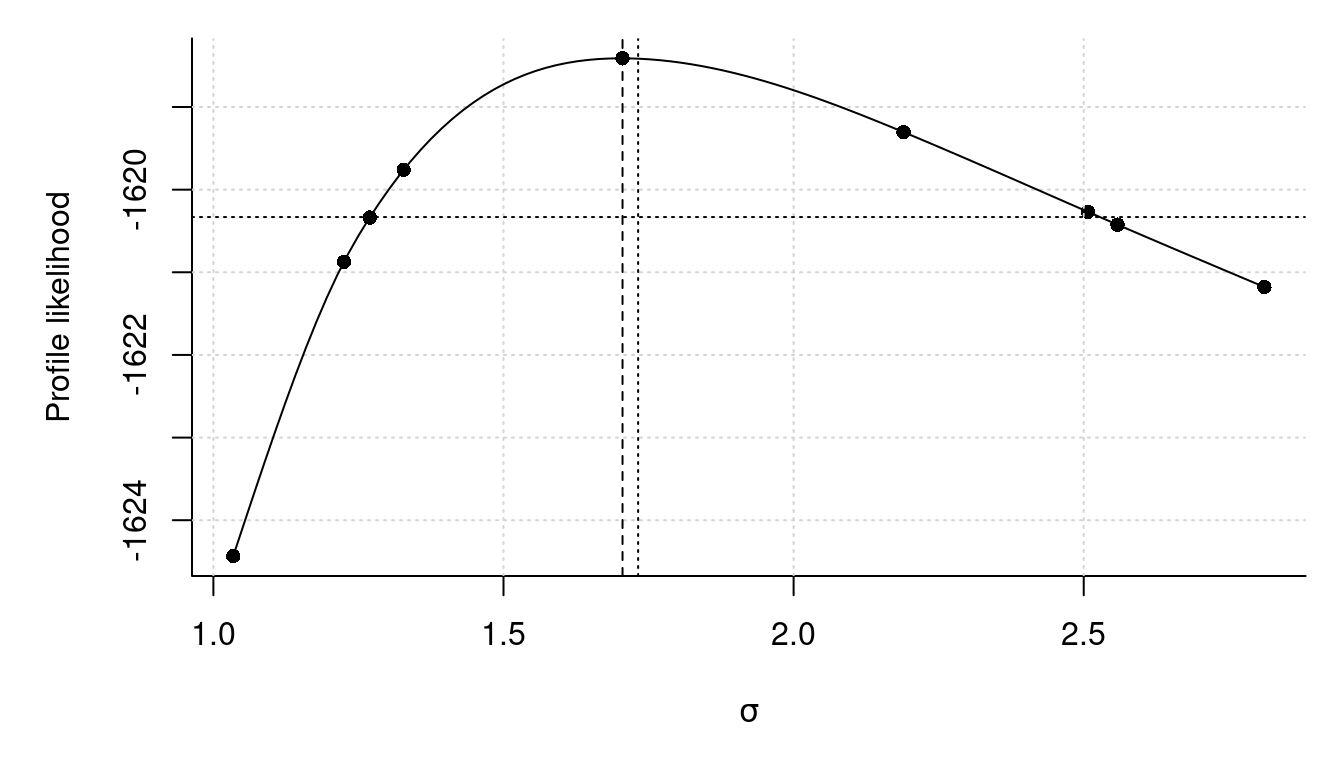# we can do the same for the slope of the binary covariates
prof_res <- pedmod_profile(
ptr = ll_terms, par = opt_out$par, delta = .5, maxvls = 10000L, minvls = 1000L, alpha = .05, abs_eps = 0, rel_eps = 1e-4, which_prof = 3L, use_aprx = TRUE, n_threads = 4L, verbose = TRUE) #> The estimate of the standard error of the log likelihood is 0.00264089. Preferably this should be below 0.001 #> #> Finding the lower limit of the profile likelihood curve #> LogLike: -1622.3662 at 1.378256 #> LogLike: -1622.3591 at 1.378256 #> LogLike: -1618.4107 at 1.878256 #> LogLike: -1619.2925 at 1.606492. Lb, target, ub: -1622.3591, -1620.3315, -1619.2925 #> LogLike: -1619.2884 at 1.606492. Lb, target, ub: -1622.3591, -1620.3315, -1619.2884 #> LogLike: -1620.4844 at 1.490049. Lb, target, ub: -1620.4844, -1620.3315, -1619.2884 #> LogLike: -1620.4816 at 1.490049. Lb, target, ub: -1620.4816, -1620.3315, -1619.2884 #> LogLike: -1620.1984 at 1.512492. Lb, target, ub: -1620.4816, -1620.3315, -1620.1984 #> LogLike: -1620.1982 at 1.512492. Lb, target, ub: -1620.4816, -1620.3315, -1620.1982 #> #> Finding the upper limit of the profile likelihood curve #> LogLike: -1619.6178 at 2.378256 #> LogLike: -1619.5991 at 2.378256 #> LogLike: -1621.3787 at 2.878256 #> LogLike: -1621.3504 at 2.878256 #> LogLike: -1620.5401 at 2.634563. Lb, target, ub: -1620.5401, -1620.3315, -1619.5991 #> LogLike: -1620.5161 at 2.634563. Lb, target, ub: -1620.5161, -1620.3315, -1619.5991 #> LogLike: -1620.2801 at 2.561453. Lb, target, ub: -1620.5161, -1620.3315, -1620.2801 #> LogLike: -1620.2571 at 2.561453. Lb, target, ub: -1620.5161, -1620.3315, -1620.2571 #> LogLike: -1618.4107 at 1.878256 # the confidence interval for the slope of the binary covariate prof_res$confs
#>  2.50 pct. 97.50 pct.
#>      1.502      2.583
# plot the estimated profile likelihood curve and check that everything looks
# fine
bin_slope <- prof_res$xs pls <- prof_res$p_log_Lik
par(mar = c(5, 5, 1, 1))
plot(bin_slope, pls, bty = "l",
pch = 16, xlab = expression(beta), ylab = "Profile likelihood")
grid()
lines(spline(bin_slope, pls, n = 100))
abline(v = opt_out$par, lty = 2) # the estimate abline(v = attr(dat, "beta"), lty = 3) # the true value abline(h = max(pls) - qchisq(.95, 1) / 2, lty = 3) # mark the critical value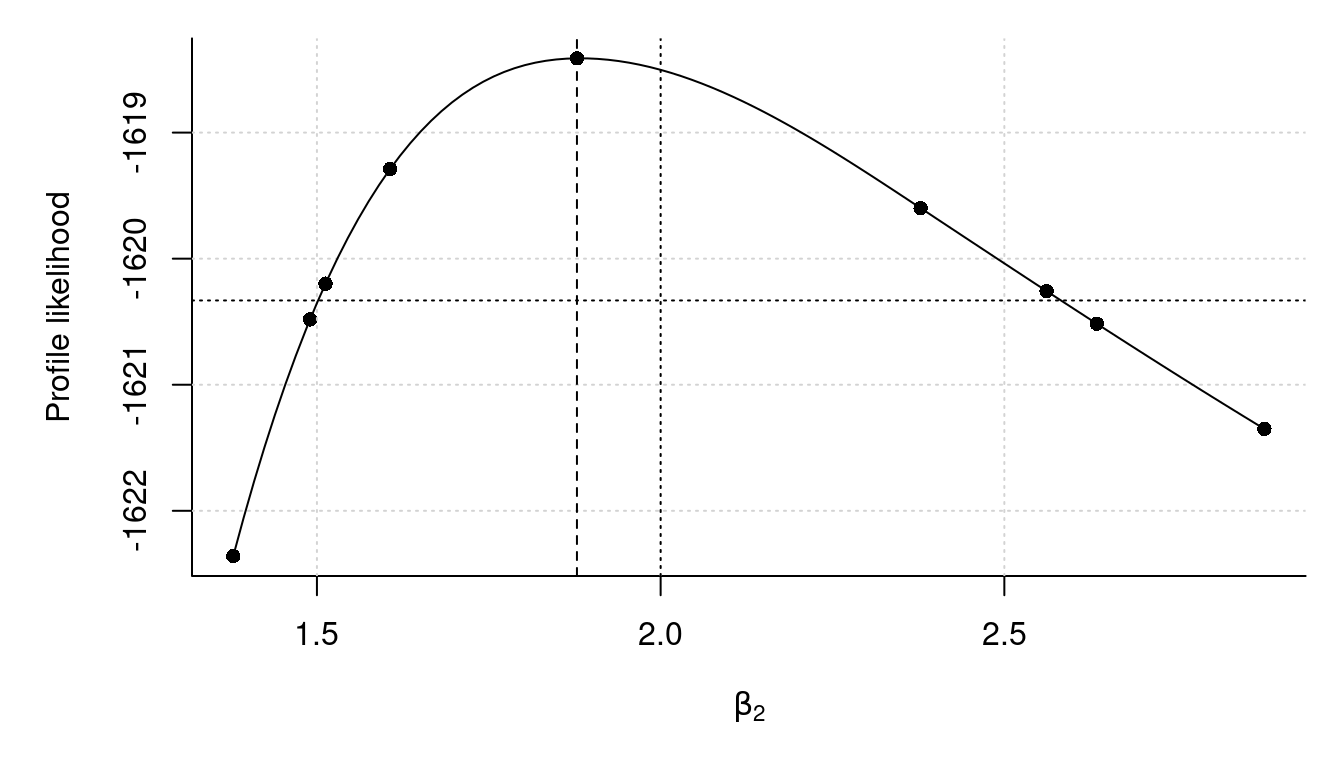We only ran the above with one seed. We can draw the curve with using different seeds to check if this does not change the estimates. We will likely need to use more samples if the result depends on the seed. # compute the profile likelihood using different seeds pl_curve_res <- lapply(1:5, function(seed) pedmod_profile( ptr = ll_terms, par = opt_out$par, delta = .5, maxvls = 10000L,
minvls = 1000L, alpha = .05, abs_eps = 0, rel_eps = 1e-4, which_prof = 4L,
use_aprx = TRUE, n_threads = 4L, seed = seed))

We show the estimated profile likelihood based confidence intervals below:

# the profile likelihood based confidence intervals
print(exp(t(sapply(pl_curve_res, [[, "confs"))), digits = 8)
#>      2.50 pct. 97.50 pct.
#> [1,] 1.6129060  6.3925594
#> [2,] 1.6113411  6.4126763
#> [3,] 1.6126743  6.3943564
#> [4,] 1.6125941  6.3911922
#> [5,] 1.6124628  6.4165030

### Randomized Quasi-Monte Carlo

There are two randomized quasi-Monte Carlo methods which are implemented in the package: randomized Korobov rules as in the implementation by Genz and Bretz (2002) and scrambled Sobol sequences. The former is used by default. The questions is which method to use. As an example, we will increase the number of samples with either methods and see how this effects the error for the gradient of the log likelihood from the first couple of families. We do this below:

# create a simple function which computes the gradient. We set the convergence
# threshold values low such that all the samples will be used
gr <- function(maxvls, method, par = start$par, minvls = 500L) eval_pedigree_grad(ptr = ll_terms, par = par, maxvls = maxvls, abs_eps = 0, rel_eps = 1e-12, indices = 0:9, minvls = minvls, method = method, n_threads = 4L) # compute the estimator for either method using an increasing number of samples n_samp <- 1000 * 2^(0:9) # the sample sizes we will use seeds <- 1:40 # the seeds we will use res <- sapply(setNames(n_samp, n_samp), function(maxvls){ sapply(c(Korobov = 0, Sobol = 1), function(method){ # estimate the gradient ests <- sapply(seeds, function(s){ set.seed(s) gr(maxvls = maxvls, minvls = maxvls, method = method) }) # return the mean of the estimators and the standard deviation rbind(mean = rowMeans(ests), sd = apply(ests, 1L, sd)) }, simplify = "array") }, simplify = "array") # set the names of the dimensions dimnames(res) <- list( metric = dimnames(res)[[1L]], parameter = names(opt_out$par),
method = dimnames(res)[[3L]], samples = n_samp)

# they seem to converge to the same estimate as expected
print(t(res["mean", , "Korobov", ]), digits = 6)
#>         parameter
#> samples  (Intercept) Continuous   Binary
#>   1000     -0.542977    3.07220 -1.64744 -0.903425
#>   2000     -0.545156    3.07124 -1.64875 -0.904159
#>   4000     -0.545396    3.07055 -1.64847 -0.903605
#>   8000     -0.545606    3.07174 -1.64928 -0.902010
#>   16000    -0.545329    3.07147 -1.64913 -0.903307
#>   32000    -0.545353    3.07142 -1.64903 -0.903075
#>   64000    -0.545338    3.07154 -1.64908 -0.902849
#>   128000   -0.545369    3.07151 -1.64908 -0.902838
#>   256000   -0.545366    3.07148 -1.64908 -0.902898
#>   512000   -0.545370    3.07149 -1.64910 -0.902875
print(t(res["mean", , "Sobol"  , ]), digits = 6)
#>         parameter
#> samples  (Intercept) Continuous   Binary
#>   1000     -0.545443    3.07244 -1.64925 -0.909546
#>   2000     -0.544713    3.07247 -1.64857 -0.907893
#>   4000     -0.545858    3.07177 -1.64887 -0.903273
#>   8000     -0.545198    3.07091 -1.64901 -0.903082
#>   16000    -0.545413    3.07152 -1.64880 -0.902484
#>   32000    -0.545362    3.07154 -1.64900 -0.902564
#>   64000    -0.545370    3.07142 -1.64907 -0.902848
#>   128000   -0.545363    3.07144 -1.64906 -0.902843
#>   256000   -0.545373    3.07149 -1.64907 -0.902815
#>   512000   -0.545372    3.07149 -1.64909 -0.902861

# get a best estimator of the gradient by combining the two
precise_est <- rowMeans(res["mean", , , length(n_samp)])

# the standard deviation of the result scaled by the absolute value of the
# estimated gradient to get the number of significant digits
round(t(res["sd", , "Korobov", ] / abs(precise_est)), 6)
#>         parameter
#> samples  (Intercept) Continuous   Binary
#>   1000      0.020412   0.008023 0.006444 0.026864
#>   2000      0.003959   0.001780 0.001473 0.007806
#>   4000      0.004619   0.002070 0.001824 0.008830
#>   8000      0.001635   0.000607 0.000610 0.003488
#>   16000     0.000653   0.000251 0.000256 0.001580
#>   32000     0.000389   0.000155 0.000168 0.001423
#>   64000     0.000235   0.000103 0.000094 0.000637
#>   128000    0.000075   0.000028 0.000025 0.000217
#>   256000    0.000046   0.000022 0.000024 0.000162
#>   512000    0.000091   0.000041 0.000033 0.000286
round(t(res["sd", , "Sobol"  , ] / abs(precise_est)), 6)
#>         parameter
#> samples  (Intercept) Continuous   Binary
#>   1000      0.019472   0.008728 0.007275 0.033470
#>   2000      0.011401   0.004239 0.004862 0.020085
#>   4000      0.006189   0.002074 0.002653 0.013707
#>   8000      0.003146   0.001051 0.001301 0.005197
#>   16000     0.001674   0.000675 0.000741 0.003351
#>   32000     0.000834   0.000346 0.000284 0.001169
#>   64000     0.000352   0.000175 0.000173 0.000862
#>   128000    0.000193   0.000083 0.000076 0.000398
#>   256000    0.000099   0.000051 0.000049 0.000203
#>   512000    0.000047   0.000020 0.000017 0.000132
# look at a log-log regression to check convergence rate. We expect a rate
# between 0.5, O(sqrt(n)) rate, and 1, O(n) rate, which can be seen from minus
# the slopes below
coef(lm(t(log(res["sd", , "Korobov", ])) ~ log(n_samp)))
#>             (Intercept) Continuous  Binary
#> (Intercept)       1.404     2.1636  1.2910  1.4868
#> log(n_samp)      -0.934    -0.9249 -0.9073 -0.8022
coef(lm(t(log(res["sd", , "Sobol", ])) ~ log(n_samp)))
#>             (Intercept) Continuous Binary
#> (Intercept)      2.3743     2.8575  2.551  3.0372
#> log(n_samp)     -0.9797    -0.9437 -0.975 -0.9277

# plot the two standard deviation estimates
par(mar = c(5, 5, 1, 1))
matplot(n_samp, t(res["sd", , "Korobov", ]), log = "xy", ylab = "L2 error",
type = "p", pch = c(0:2, 5L), col = "black", bty = "l",
xlab = "Number of samples", ylim = range(res["sd", , , ]))
matlines(n_samp, t(res["sd", , "Korobov", ]), col = "black", lty = 2)

# add the points from Sobol method
matplot(n_samp, t(res["sd", , "Sobol", ]), type = "p", pch = 15:18,
col = "darkgray", add = TRUE)
matlines(n_samp, t(res["sd", , "Sobol", ]), col = "darkgray", lty = 3)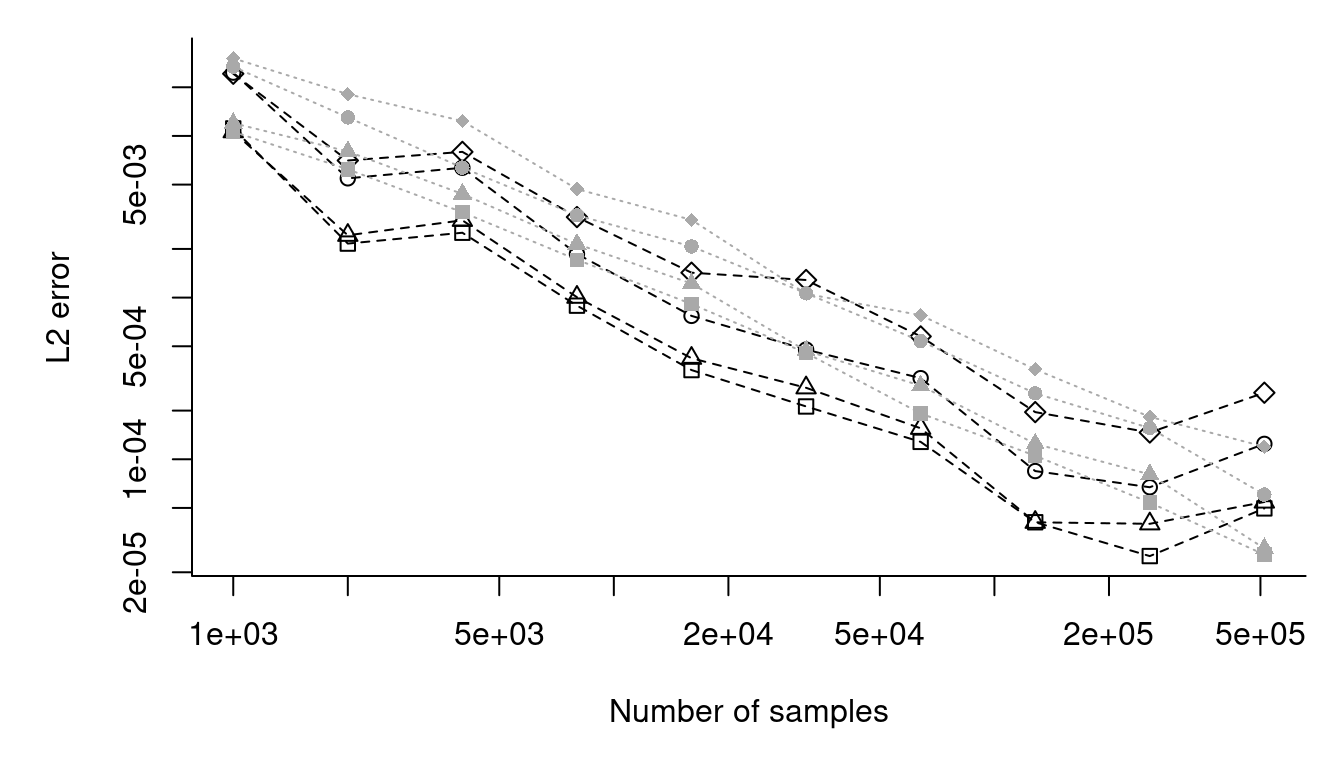The above seems to suggest that the randomized Korobov rules are preferable and that both method achieve close to a $$O(n^{-1 + \epsilon})$$ rate for some small $$\epsilon$$. Notice that we have to set minvls equal to maxvls to achieve the $$O(n^{-1 + \epsilon})$$ rate with randomized Korobov rules.

We can also consider the convergence rate for the log likelihood. This time, we also consider the error using the minimax tilted version suggested by Botev (2017). We also show how the error can be reduced by using fewer randomized qausi-Monte Carlo sequences at the cost of the precision of the error estimate:

# create a simple function which computes the log likelihood. We set the
# convergence threshold values low such that all the samples will be used
fn <- function(maxvls, method, par = start$par, ptr = ll_terms, minvls = 500L, use_tilting) eval_pedigree_ll(ptr = ptr, par = par, maxvls = maxvls, abs_eps = 0, rel_eps = 1e-12, indices = 0:9, minvls = minvls, method = method, n_threads = 4L, use_tilting = use_tilting) # compute the estimator for either method using an increasing number of samples res <- sapply(setNames(n_samp, n_samp), function(maxvls){ sapply(c(W/ tilting = TRUE, W/o tilting = FALSE), function(use_tilting){ sapply(c(Korobov = 0, Sobol = 1), function(method){ # estimate the gradient ests <- sapply(seeds, function(s){ set.seed(s) fn(maxvls = maxvls, minvls = maxvls, method = method, use_tilting = use_tilting) }) # return the mean of the estimators and the standard deviation c(mean = mean(ests), sd = sd(ests)) }, simplify = "array") }, simplify = "array") }, simplify = "array") # compute the errors with fewer randomized quasi-Monte Carlo sequences ll_terms_few_sequences <- pedigree_ll_terms(dat, max_threads = 4L, n_sequences = 1L) res_few_seqs <- sapply(setNames(n_samp, n_samp), function(maxvls){ sapply(c(W/ tilting = TRUE, W/o tilting = FALSE), function(use_tilting){ sapply(c(Korobov = 0, Sobol = 1), function(method){ # estimate the gradient ests <- sapply(seeds, function(s){ set.seed(s) fn(maxvls = maxvls, minvls = maxvls, method = method, ptr = ll_terms_few_sequences, use_tilting = use_tilting) }) # return the mean of the estimators and the standard deviation c(mean = mean(ests), sd = sd(ests)) }, simplify = "array") }, simplify = "array") }, simplify = "array") # the standard deviation of the result scaled by the absolute value of the # estimated log likelihood to get the number of significant digits. Notice that # we scale up the figures by 1000! precise_est <- mean(res["mean", , , length(n_samp)]) round(1000 * res["sd", "Korobov", , ] / abs(precise_est), 6) #> 1000 2000 4000 8000 16000 32000 64000 #> W/ tilting 0.05252 0.008957 0.01149 0.004568 0.001833 0.001027 0.000574 #> W/o tilting 0.06358 0.011445 0.01383 0.004873 0.002329 0.000949 0.000855 #> 128000 256000 512000 #> W/ tilting 0.000190 0.000102 0.000123 #> W/o tilting 0.000219 0.000160 0.000245 round(1000 * res["sd", "Sobol" , , ] / abs(precise_est), 6) #> 1000 2000 4000 8000 16000 32000 64000 #> W/ tilting 0.03416 0.02132 0.01507 0.006704 0.003523 0.002073 0.001081 #> W/o tilting 0.10916 0.04650 0.02482 0.011072 0.006090 0.003202 0.001260 #> 128000 256000 512000 #> W/ tilting 0.000479 0.000238 0.000101 #> W/o tilting 0.000630 0.000336 0.000170 # with fewer sequences round(1000 * res_few_seqs["sd", "Korobov", , ] / abs(precise_est), 6) #> 1000 2000 4000 8000 16000 32000 64000 #> W/ tilting 0.01134 0.005193 0.002952 0.001582 0.000506 0.000354 0.000412 #> W/o tilting 0.01390 0.004954 0.003055 0.002181 0.000653 0.000439 0.000625 #> 128000 256000 512000 #> W/ tilting 0.000223 4.8e-05 5.1e-05 #> W/o tilting 0.000190 5.1e-05 5.3e-05 round(1000 * res_few_seqs["sd", "Sobol" , , ] / abs(precise_est), 6) #> 1000 2000 4000 8000 16000 32000 64000 #> W/ tilting 0.01701 0.008483 0.005322 0.002887 0.001269 0.000766 0.000323 #> W/o tilting 0.03370 0.016389 0.007411 0.004601 0.001951 0.000921 0.000505 #> 128000 256000 512000 #> W/ tilting 0.000130 0.000066 3.0e-05 #> W/o tilting 0.000208 0.000109 6.3e-05 # look at log-log regressions apply(res["sd", , , ], 1:2, function(sds) coef(lm(log(sds) ~ log(n_samp)))) #> , , W/ tilting #> #> Korobov Sobol #> (Intercept) 0.1604 0.1002 #> log(n_samp) -0.9890 -0.9366 #> #> , , W/o tilting #> #> Korobov Sobol #> (Intercept) -0.1441 1.593 #> log(n_samp) -0.9335 -1.033 # plot the standard deviation estimates. Dashed lines are with fewer sequences par(mar = c(5, 5, 1, 1)) create_plot <- function(results, ylim){ sds <- matrix(results["sd", , , ], ncol = dim(results)) dimnames(sds) <- list(do.call(outer, c(dimnames(results)[2:3], list(FUN = paste))), NULL) lty <- c(1, 1, 2, 2) col <- rep(c("black", "darkgray"), 2) matplot(n_samp, t(sds), log = "xy", ylab = "L2 error", lty = lty, type = "l", bty = "l", xlab = "Number of samples", col = col, ylim = ylim) matplot(n_samp, t(sds), pch = c(1, 16), col = col, add = TRUE) legend("bottomleft", bty = "n", lty = lty, col = col, legend = rownames(sds)) grid() } # with more sequences ylim_plot <- range(res["sd", , , ], res_few_seqs["sd", , , ]) create_plot(res, ylim = ylim_plot)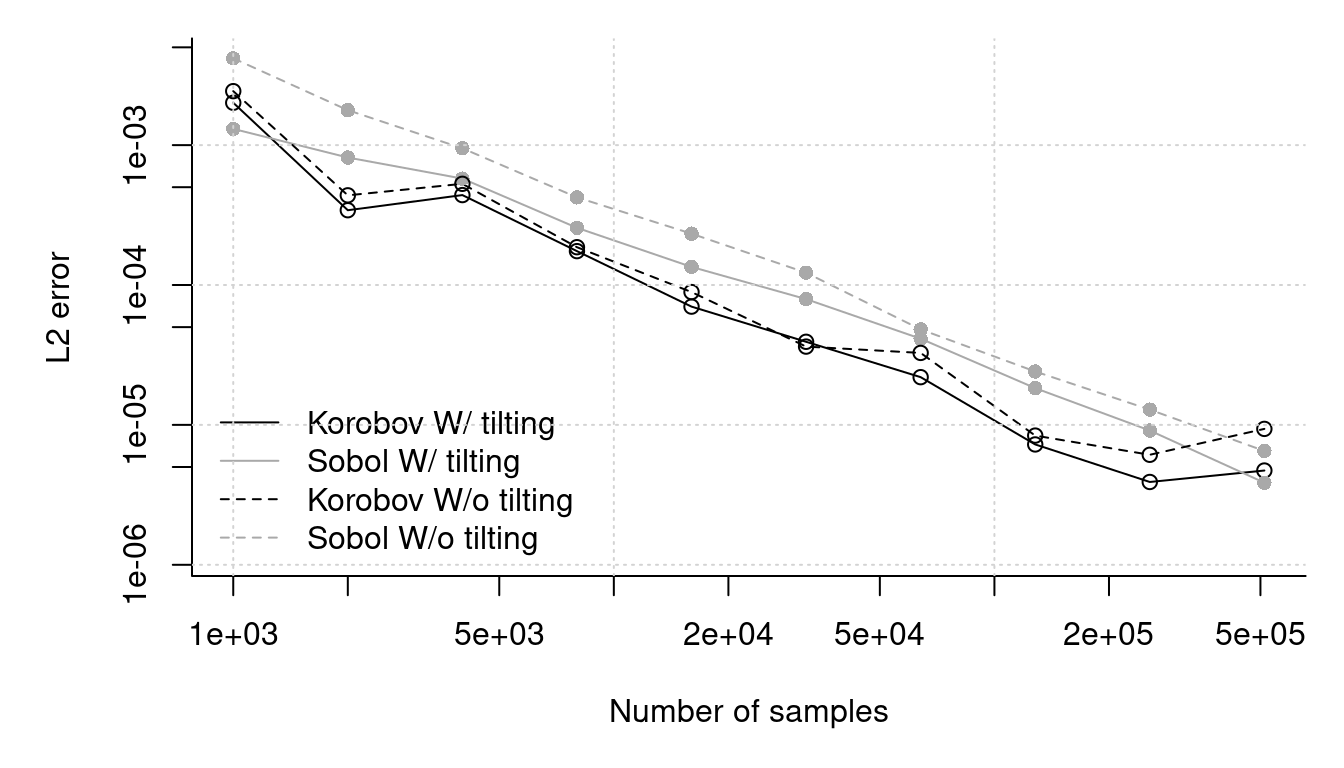# with one sequence create_plot(res_few_seqs, ylim = ylim_plot)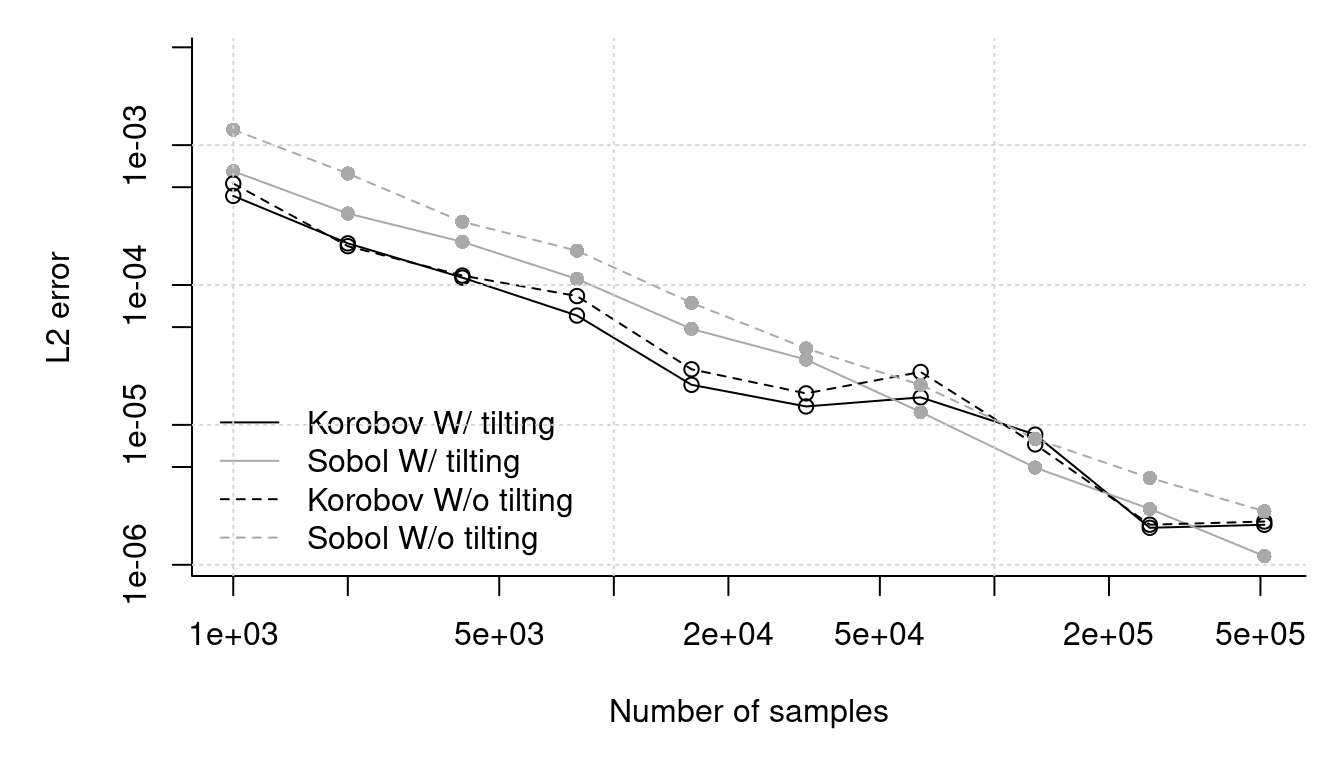Again the randomized Korobov rules seems preferable. In general, a strategy can be to use only one randomized quasi-Monte Carlo sequence as above and set minvls and maxvls to the desired number of samples. This will though imply that the method cannot stop early if it is easy to approximate the log likelihood and its derivative. We fit the model again below as example of using the scrambled Sobol sequences: # estimate the model using Sobol sequences system.time( opt_out_sobol <- pedmod_opt( ptr = ll_terms, par = start$par, abs_eps = 0, use_aprx = TRUE,
maxvls = 25000L, rel_eps = 1e-3, minvls = 5000L, method = 1L))
#>    user  system elapsed
#>  48.004   0.008  12.046

print(-opt_out_sobol$value, digits = 8) #>  -1618.4027 print(-opt_out$value, digits = 8)
#>  -1618.4045

# the parameters
rbind(Korobov = opt_out      $par, Sobol = opt_out_sobol$par)
#>         (Intercept) Continuous Binary
#> Korobov      -2.872     0.9689  1.878 1.067
#> Sobol        -2.874     0.9692  1.880 1.068

# number of used function and gradient evaluations
opt_out$counts #> function gradient #> 37 12 opt_out_sobol$counts
#>       12       10

### Simulation Study

We make a small simulation study below where we are interested in the estimation time and bias.

# the seeds we will use
seeds <- c(36451989L, 18774630L, 76585289L, 31898455L, 55733878L, 99681114L, 37725150L, 99188448L, 66989159L, 20673587L, 47985954L, 42571905L, 53089211L, 18457743L, 96049437L, 70222325L, 86393368L, 45380572L, 81116968L, 48291155L, 89755299L, 69891073L, 1846862L, 15263013L, 37537710L,
25194071L, 14471551L, 38278606L, 55596031L, 5436537L, 75008107L, 83382936L, 50689482L, 71708788L, 52258337L, 23423931L, 61069524L, 24452554L, 32406673L, 14900280L, 24818537L, 59733700L, 82407492L, 95500692L, 62528680L, 88728797L, 9891891L, 36354594L, 69630736L, 41755287L)

# run the simulation study
sim_study <- lapply(seeds, function(s){
set.seed(s)

# only run the result if it has not been computed
f <- file.path("cache", "sim_study_simple", paste0("simple-", s, ".RDS"))
if(!file.exists(f)){
# simulate the data
dat <- sim_dat(n_fams = 400L)

# get the starting values
library(pedmod)
do_fit <- function(standardized){
ll_terms <- pedigree_ll_terms(dat, max_threads = 4L)
ti_start <- system.time(start <- pedmod_start(
ptr = ll_terms, data = dat, n_threads = 4L,
standardized = standardized))
start$time <- ti_start ti_fit <- system.time( opt_out <- pedmod_opt( ptr = ll_terms, par = start$par, abs_eps = 0, use_aprx = TRUE,
maxvls = 25000L, rel_eps = 1e-3, minvls = 5000L,
standardized = standardized))
opt_out$time <- ti_fit if(standardized){ start$par   <- standardized_to_direct(start$par, 1L) opt_out$par <- standardized_to_direct(opt_out$par, 1L) } list(start = start, opt_out = opt_out, ll_no_rng = start$logLik_no_rng)
}

fit_direct <- do_fit(standardized = FALSE)
fit_std    <- do_fit(standardized = TRUE)
saveRDS(list(fit_direct = fit_direct, fit_std = fit_std), f)
}

# report to console and return
message(paste0(capture.output(out$fit_direct$opt_out$par), collapse = "\n")) message(paste0(capture.output(out$fit_std   $opt_out$par), collapse = "\n"))
message(sprintf(
"Time %12.1f, %12.1f. Max ll: %12.4f, %12.4f\n",
with(out$fit_direct, start$time["elapsed"] + opt_out$time["elapsed"]), with(out$fit_std   , start$time["elapsed"] + opt_out$time["elapsed"]),
-out$fit_direct$opt_out$value, -out$fit_std   $opt_out$value))

out
})

# gather the estimates
beta_est <- sapply(sim_study, function(x)
cbind(Direct       = head(x$fit_direct$opt_out$par, 3), Standardized = head(x$fit_std   $opt_out$par, 3)),
simplify = "array")
sigma_est <- sapply(sim_study, function(x)
cbind(Direct       = exp(tail(x$fit_direct$opt_out$par, 1) / 2), Standardized = exp(tail(x$fit_std   $opt_out$par, 1) / 2)),
simplify = "array")

# compute the errors
tmp <- sim_dat(2L)
err_beta  <- beta_est  - attr(tmp, "beta")
err_sigma <- sigma_est - sqrt(attr(tmp, "sig_sq"))
dimnames(err_sigma)[[1L]] <- "std genetic"
err <- abind::abind(err_beta, err_sigma, along = 1)

# get the bias estimates and the standard errors
bias <- apply(err, 1:2, mean)
n_sims <- dim(err)[]
SE <- apply(err , 1:2, sd) / sqrt(n_sims)
bias
#>               Direct Standardized
#> (Intercept) -0.06567     -0.06507
#> Continuous   0.02814      0.02796
#> Binary       0.03732      0.03677
#> std genetic  0.05619      0.05587
SE
#>              Direct Standardized
#> (Intercept) 0.05068      0.05024
#> Continuous  0.01714      0.01703
#> Binary      0.03360      0.03327
#> std genetic 0.03900      0.03868

# make a box plot
b_vals <- expand.grid(rownames(err), strtrim(colnames(err), 1))
box_dat <- data.frame(Error = c(err),
Parameter = rep(b_vals$Var1, n_sims), Method = rep(b_vals$Var2, dim(err)[]))
par(mar = c(7, 5, 1, 1))
# S is for the standardized and D is for the direct parameterization
boxplot(Error ~ Method + Parameter, box_dat, ylab = "Error", las = 2,
xlab = "")
abline(h = 0, lty = 2)
grid()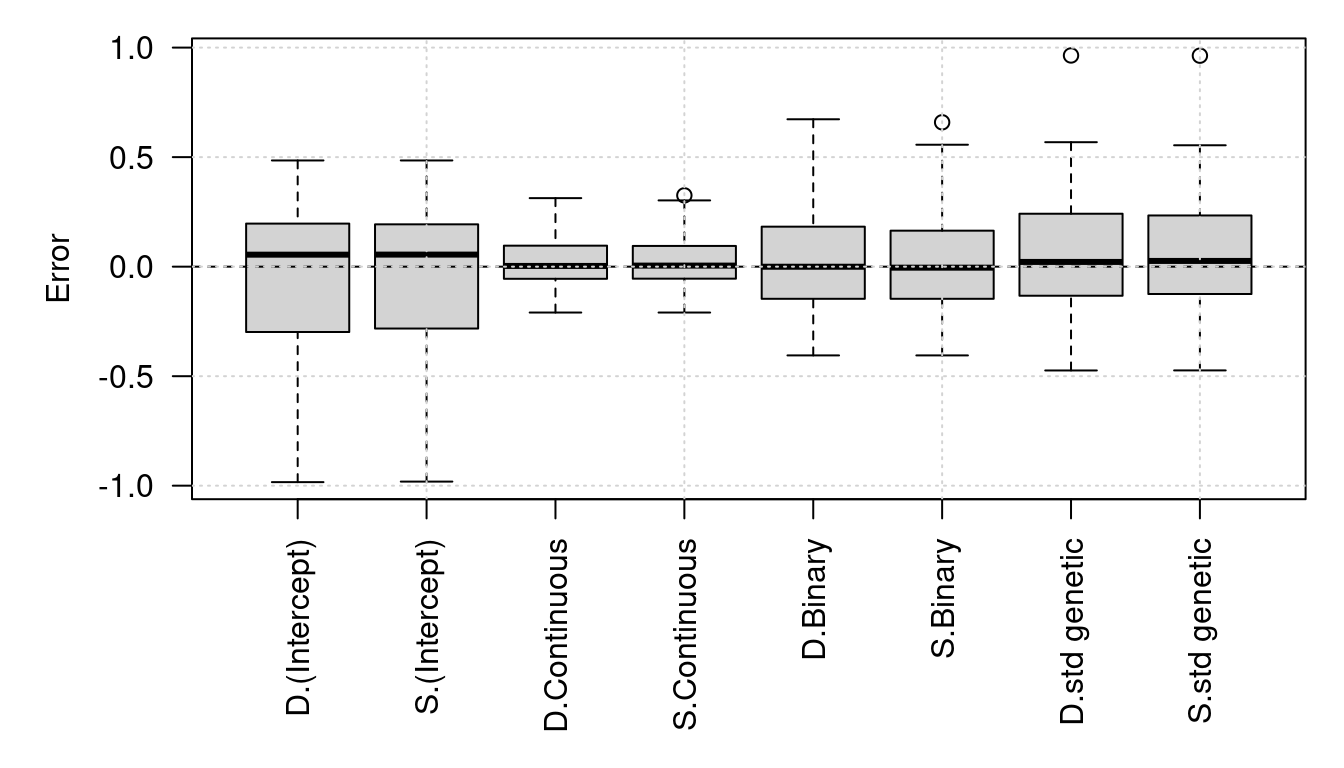# get the average computation times
time_vals <- sapply(sim_study, function(x) {
. <- function(z){
keep <- c("opt_out", "start")
out <- setNames(sapply(z[keep], function(z) z$time["elapsed"]), keep) c(out, total = sum(out)) } rbind(Direct = .(x$fit_direct),
Standardized = .(xfit_std)) }, simplify = "array") apply(time_vals, 1:2, mean) #> opt_out start total #> Direct 5.709 1.809 7.518 #> Standardized 7.464 1.818 9.282 apply(time_vals, 1:2, sd) #> opt_out start total #> Direct 3.201 1.03 3.499 #> Standardized 2.294 1.18 2.491 apply(time_vals, 1:2, quantile) #> , , opt_out #> #> Direct Standardized #> 0% 2.540 3.746 #> 25% 3.779 5.703 #> 50% 4.145 7.796 #> 75% 7.588 8.910 #> 100% 20.125 12.145 #> #> , , start #> #> Direct Standardized #> 0% 0.680 0.726 #> 25% 1.170 1.105 #> 50% 1.447 1.332 #> 75% 2.019 1.993 #> 100% 5.776 6.279 #> #> , , total #> #> Direct Standardized #> 0% 3.759 5.069 #> 25% 5.157 7.216 #> 50% 6.336 9.308 #> 75% 9.122 10.950 #> 100% 23.930 14.392 ## Example: Adding Child Environment Effects As an extension, we can add a child environment effect. The new scale matrix, the $$C_{i2}$$’s, can be written as: C_env <- diag(1, NROW(fam)) C_env[c(3, 5), c(3, 5)] <- 1 C_env[c(7:8 ), c(7:8 )] <- 1 C_env[c(9:10), c(9:10)] <- 1 Matrix::Matrix(C_env, sparse = TRUE) #> 10 x 10 sparse Matrix of class "dsCMatrix" #> #> [1,] 1 . . . . . . . . . #> [2,] . 1 . . . . . . . . #> [3,] . . 1 . 1 . . . . . #> [4,] . . . 1 . . . . . . #> [5,] . . 1 . 1 . . . . . #> [6,] . . . . . 1 . . . . #> [7,] . . . . . . 1 1 . . #> [8,] . . . . . . 1 1 . . #> [9,] . . . . . . . . 1 1 #> [10,] . . . . . . . . 1 1 We assign the new simulation function below but this time we include only binary covariates: # simulates a data set. # # Args: # n_fams: number of families. # beta: the fixed effect coefficients. # sig_sq: the scale parameters. sim_dat <- function(n_fams, beta = c(-3, 4), sig_sq = c(2, 1)){ # setup before the simulations Cmat <- 2 * kinship(ped) n_obs <- NROW(fam) Sig <- diag(n_obs) + sig_sq * Cmat + sig_sq * C_env Sig_chol <- chol(Sig) # simulate the data out <- replicate( n_fams, { # simulate covariates X <- cbind((Intercept) = 1, Binary = runif(n_obs) > .9) # assign the linear predictor + noise eta <- drop(X %*% beta) + drop(rnorm(n_obs) %*% Sig_chol) # return the list in the format needed for the package list(y = as.numeric(eta > 0), X = X, scale_mats = list( Genetic = Cmat, Environment = C_env)) }, simplify = FALSE) # add attributes with the true values and return attributes(out) <- list(beta = beta, sig_sq = sig_sq) out } The model is \begin{align*} Y_{ij} &= \begin{cases} 1 & \beta_0 + \beta_1 B_{ij} + E_{ij} + G_{ij} + R_{ij} > 0 \\ 0 & \text{otherwise} \end{cases} \\ X_{ij} &\sim N(0, 1) \\ B_{ij} &\sim \text{Bin}(0.1, 1) \\ (G_{i1}, \dots, G_{in_{i}})^\top &\sim N^{(n_i)}(\vec 0, \sigma^2_G C_{i1}) \\ (E_{i1}, \dots, E_{in_{i}})^\top &\sim N^{(n_i)}(\vec 0, \sigma^2_E C_{i2}) \\ R_{ij} &\sim N(0, 1)\end{align*} where $$C_{i1}$$ is two times the kinship matrix, $$C_{i2}$$ is singular matrix for the environment effect, and $$B_{ij}$$ is an observed covariate. In this case, we exploit that some of log marginal likelihood terms are identical. That is, some of the combinations of pedigrees, covariates, and outcomes match. Therefor, we can use the cluster_weights arguments to reduce the computation time as shown below: # simulate a data set set.seed(27107390) dat <- sim_dat(n_fams = 1000L) # compute the log marginal likelihood by not using that some of the log marginal # likelihood terms are identical beta_true <- attr(dat, "beta") sig_sq_true <- attr(dat, "sig_sq") library(pedmod) ll_terms_wo_weights <- pedigree_ll_terms(dat, max_threads = 4L) system.time(ll_res <- eval_pedigree_ll( ll_terms_wo_weights, c(beta_true, log(sig_sq_true)), maxvls = 100000L, abs_eps = 0, rel_eps = 1e-3, minvls = 2500L, use_aprx = TRUE, n_threads = 4)) #> user system elapsed #> 0.588 0.000 0.150 system.time(grad_res <- eval_pedigree_grad( ll_terms_wo_weights, c(beta_true, log(sig_sq_true)), maxvls = 100000L, abs_eps = 0, rel_eps = 1e-3, minvls = 2500L, use_aprx = TRUE, n_threads = 4)) #> user system elapsed #> 14.426 0.000 3.668 # find the duplicated combinations of pedigrees, covariates, and outcomes. One # likely needs to change this code if the pedigrees are not identical but are # identical if they are permuted. In this case, the code below will miss # identical log marginal likelihood terms dat_unqiue <- dat[!duplicated(dat)] attributes(dat_unqiue) <- attributes(dat) length(dat_unqiue) # number of unique terms #>  420 # get the weights. This can be written in a much more efficient way c_weights <- sapply(dat_unqiue, function(x) sum(sapply(dat, identical, y = x))) # get the C++ object and show that the computation time is reduced ll_terms <- pedigree_ll_terms(dat_unqiue, max_threads = 4L) system.time(ll_res_fast <- eval_pedigree_ll( ll_terms, c(beta_true, log(sig_sq_true)), maxvls = 100000L, abs_eps = 0, rel_eps = 1e-3, minvls = 2500L, use_aprx = TRUE, n_threads = 4, cluster_weights = c_weights)) #> user system elapsed #> 0.241 0.000 0.062 system.time(grad_res_fast <- eval_pedigree_grad( ll_terms, c(beta_true, log(sig_sq_true)), maxvls = 100000L, abs_eps = 0, rel_eps = 1e-3, minvls = 2500L, use_aprx = TRUE, n_threads = 4, cluster_weights = c_weights)) #> user system elapsed #> 6.104 0.000 1.598 # show that we get the same (up to a Monte Carlo error) print(c(redundant = ll_res, fast = ll_res_fast), digits = 6) #> redundant fast #> -2696.62 -2696.63 rbind(redundant = grad_res, fast = grad_res_fast) #> [,1] [,2] [,3] [,4] #> redundant -12.03 5.148 -13.48 -8.580 #> fast -12.05 5.155 -13.56 -8.665 rm(dat) # will not need this anymore # note that the variance is greater for the weighted version ll_ests <- sapply(1:50, function(seed){ set.seed(seed) eval_pedigree_ll( ll_terms_wo_weights, c(beta_true, log(sig_sq_true)), maxvls = 100000L, abs_eps = 0, rel_eps = 1e-3, minvls = 2500L, use_aprx = TRUE, n_threads = 4) }) ll_ests_fast <- sapply(1:50, function(seed){ set.seed(seed) eval_pedigree_ll( ll_terms, c(beta_true, log(sig_sq_true)), maxvls = 10000L, abs_eps = 0, rel_eps = 1e-3, minvls = 2500L, use_aprx = TRUE, n_threads = 4, cluster_weights = c_weights) }) # the estimates are comparable c(Without weights = mean(ll_ests), With weights = mean(ll_ests_fast)) #> Without weights With weights #> -2697 -2697 # the standard deviation is different c(Without weights = sd(ll_ests), With weights = sd(ll_ests_fast)) #> Without weights With weights #> 0.003629 0.020053 # we can mitigate this by using the vls_scales argument which though is a bit # slower ll_ests_fast_vls_scales <- sapply(1:50, function(seed){ set.seed(seed) eval_pedigree_ll( ll_terms, c(beta_true, log(sig_sq_true)), maxvls = 10000L, abs_eps = 0, rel_eps = 1e-3, minvls = 2500L, use_aprx = TRUE, n_threads = 4, cluster_weights = c_weights, vls_scales = sqrt(c_weights)) }) # the estimates are comparable c(Without weights = mean(ll_ests), With weights = mean(ll_ests_fast), With weights and vls_scales = mean(ll_ests_fast_vls_scales)) #> Without weights With weights #> -2697 -2697 #> With weights and vls_scales #> -2697 # the standard deviation is different c(Without weights = sd(ll_ests), With weights = sd(ll_ests_fast), With weights and vls_scales = sd(ll_ests_fast_vls_scales)) #> Without weights With weights #> 0.003629 0.020053 #> With weights and vls_scales #> 0.004966 # it is still faster system.time(ll_res_fast <- eval_pedigree_ll( ll_terms, c(beta_true, log(sig_sq_true)), maxvls = 100000L, abs_eps = 0, rel_eps = 1e-3, minvls = 2500L, use_aprx = TRUE, n_threads = 4, cluster_weights = c_weights, vls_scales = sqrt(c_weights))) #> user system elapsed #> 0.364 0.000 0.122 system.time(grad_res_fast <- eval_pedigree_grad( ll_terms, c(beta_true, log(sig_sq_true)), maxvls = 100000L, abs_eps = 0, rel_eps = 1e-3, minvls = 2500L, use_aprx = TRUE, n_threads = 4, cluster_weights = c_weights, vls_scales = sqrt(c_weights))) #> user system elapsed #> 6.435 0.000 1.696 # find the starting values system.time(start <- pedmod_start( ptr = ll_terms, data = dat_unqiue, cluster_weights = c_weights, vls_scales = sqrt(c_weights))) #> user system elapsed #> 7.393 0.000 7.392 # optimize system.time( opt_out_quick <- pedmod_opt( ptr = ll_terms, par = startpar, abs_eps = 0, use_aprx = TRUE,
n_threads = 4L,  cluster_weights = c_weights,
maxvls = 5000L, rel_eps = 1e-2, minvls = 500L,
vls_scales = sqrt(c_weights)))
#>    user  system elapsed
#>   6.423   0.000   1.688
system.time(
opt_out <- pedmod_opt(
ptr = ll_terms, par = opt_out_quick$par, abs_eps = 0, use_aprx = TRUE, n_threads = 4L, cluster_weights = c_weights, vls_scales = sqrt(c_weights), # we changed these parameters maxvls = 25000L, rel_eps = 1e-3, minvls = 5000L)) #> user system elapsed #> 32.58 0.00 10.23 The results are shown below: # parameter estimates versus the truth rbind(opt_out = head(opt_out$par, -2),
opt_out_quick = head(start  $par, -2), truth = attr(dat_unqiue, "beta")) #> (Intercept) Binary #> opt_out -2.919 3.907 #> opt_out_quick -2.919 3.900 #> truth -3.000 4.000 rbind(opt_out = exp(tail(opt_out$par, 2)),
opt_out_quick = exp(tail(start  $par, 2)), truth = attr(dat_unqiue, "sig_sq")) #> #> opt_out 1.853 0.8399 #> opt_out_quick 1.838 0.8650 #> truth 2.000 1.0000 # log marginal likelihoods print( start$logLik_est, digits = 8)  # this is unreliably/imprecise
#>  -2696.1413
print(-opt_out$value , digits = 8) #>  -2696.1125 ### Motivation of Different Number of Samples We use the cluster_weights argument above to exploit that some of the log marginal likelihood terms are identical. Specifically, let $$l_j$$ be the $$j$$th distinct log marginal likelihood term and $$\vec\theta$$ be the model parameters, then we use that the log marginal likelihood is $l(\vec\theta) = \sum_{j = 1}^L\sum_{i = 1}^{w_j}l_j(\vec\theta) = \sum_{j = 1}^Lw_jl_j(\vec\theta).$ The unweighted version is the left hand side and the weighted version is the right hand side. The two have different variances. Our quasi-Monte-Carlo method has (almost) a variance for each $$\exp l_j$$ which is $$\mathcal{O}(m^{-2})$$ with $$m$$ being the number of samples we use for each $$l_j$$. Thus, the variance of the unweighted version is $\sum_{l = j}^L\sum_{i = 1}^{w_j}\text{Var}(l_j(\vec\theta))$ which is $\mathcal{O}\left(\sum_{j = 1}^L \frac{w_j}{m^2}\right)$ However, the variance of the weighted version is $\sum_{j = 1}^L\text{Var}(w_jl_j(\vec\theta))$ which is $\mathcal{O}\left(\sum_{j = 1}^L \frac{w_j^2}{m^2}\right)$ Though, we can get a similar variance by using $$\sqrt{w_j}m$$ samples for term $$j$$. The variance then becomes $\mathcal{O}\left(\sum_{j = 1}^L \frac{w_j^2}{w_jm^2}\right) = \mathcal{O}\left(\sum_{j = 1}^L \frac{w_j}{m^2}\right)$ but we do so using only $m\sum_{j = 1}^L\sqrt{w_j}$ samples rather than $m\sum_{j = 1}^Lw_j.$ ### Alternative Parameterization As before, we can also work with the standardized parameterization. ##### # transform the parameters and check that we get the same likelihood std_par <- direct_to_standardized(opt_out$par, n_scales = 2L)
std_par # the standardized parameterization
#> (Intercept)      Binary
#>     -1.5189      2.0334      0.6166     -0.1745
opt_out$par # the direct parameterization #> (Intercept) Binary #> -2.9187 3.9073 0.6166 -0.1745 # we can map back as follows par_back <- standardized_to_direct(std_par, n_scales = 2L) all.equal(opt_out$par, par_back, check.attributes = FALSE)
#>  TRUE
# the proportion of variance of each effect
attr(par_back, "variance proportions")
#> Residual
#>   0.2708   0.5017   0.2275

# the proportions match
total_var <- sum(exp(tail(opt_out$par, 2))) + 1 exp(tail(opt_out$par, 2)) / total_var
#>
#> 0.5017 0.2275

# compute the likelihood with either parameterization
set.seed(1L)
eval_pedigree_ll(ptr = ll_terms, par = opt_out$par, maxvls = 10000L, minvls = 1000L, rel_eps = 1e-3, use_aprx = TRUE, abs_eps = 0, cluster_weights = c_weights, vls_scales = sqrt(c_weights)) #>  -2696 #> attr(,"n_fails") #>  2 #> attr(,"std") #>  0.008541 set.seed(1L) eval_pedigree_ll(ptr = ll_terms, par = std_par , maxvls = 10000L, minvls = 1000L, rel_eps = 1e-3, use_aprx = TRUE, abs_eps = 0, cluster_weights = c_weights, vls_scales = sqrt(c_weights), standardized = TRUE) #>  -2696 #> attr(,"n_fails") #>  2 #> attr(,"std") #>  0.008541 # we can also get the same gradient with an application of the chain rule jac <- attr( standardized_to_direct(std_par, n_scales = 2L, jacobian = TRUE), "jacobian") set.seed(1L) g1 <- eval_pedigree_grad(ptr = ll_terms, par = opt_out$par, maxvls = 10000L,
minvls = 1000L, rel_eps = 1e-3, use_aprx = TRUE,
abs_eps = 0, cluster_weights = c_weights,
vls_scales = sqrt(c_weights))
set.seed(1L)
g2 <- eval_pedigree_grad(ptr = ll_terms, par = std_par, maxvls = 10000L,
minvls = 1000L, rel_eps = 1e-3, use_aprx = TRUE,
abs_eps = 0, standardized = TRUE,
cluster_weights = c_weights,
vls_scales = sqrt(c_weights))
all.equal(drop(g1 %*% jac), g2, check.attributes = FALSE)
#>  TRUE

The model can also be estimated with the the standardized parameterization:

# perform the optimization. We start with finding the starting values
system.time(start_std <- pedmod_start(
ptr = ll_terms, data = dat_unqiue, cluster_weights = c_weights,
vls_scales = sqrt(c_weights), standardized = TRUE))
#>    user  system elapsed
#>   7.119   0.004   7.124

# are the starting values similar?
standardized_to_direct(start_std$par, n_scales = 2L) #> (Intercept) Binary #> -2.9192 3.8995 0.6088 -0.1450 #> attr(,"variance proportions") #> Residual #> 0.2700 0.4964 0.2336 start$par
#> (Intercept)      Binary
#>     -2.9192      3.8995      0.6088     -0.1450

# this may have required different number of gradient and function evaluations
start_std$opt$counts
#>       38       38
start    $opt$counts
#>       40       40

# estimate the model
system.time(
opt_out_quick_std <- pedmod_opt(
ptr = ll_terms, par = start_std$par, abs_eps = 0, use_aprx = TRUE, n_threads = 4L, cluster_weights = c_weights, standardized = TRUE, maxvls = 5000L, rel_eps = 1e-2, minvls = 500L, vls_scales = sqrt(c_weights))) #> user system elapsed #> 6.107 0.000 1.606 system.time( opt_out_std <- pedmod_opt( ptr = ll_terms, par = opt_out_quick_std$par, abs_eps = 0, use_aprx = TRUE,
n_threads = 4L,  cluster_weights = c_weights, standardized = TRUE,
vls_scales = sqrt(c_weights),
# we changed these parameters
maxvls = 25000L, rel_eps = 1e-3, minvls = 5000L))
#>    user  system elapsed
#>   5.459   0.000   1.727

# we get the same
standardized_to_direct(opt_out_std$par, n_scales = 2L) #> (Intercept) Binary #> -2.9223 3.9117 0.6162 -0.1625 #> attr(,"variance proportions") #> Residual #> 0.2701 0.5002 0.2296 opt_out$par
#> (Intercept)      Binary
#>     -2.9187      3.9073      0.6166     -0.1745

# this may have required different number of gradient and function evaluations
opt_out_quick_std$counts #> function gradient #> 22 8 opt_out_quick$counts
#>       23        8

opt_out_std$counts #> function gradient #> 4 1 opt_out$counts
#>       22        6

### Profile Likelihood Curve

We can make a 2D profile likelihood curve as follows:

# get the values at which we evaluate the profile likelihood
rg <- Map(function(est, truth)
range(exp(est / 2) * c(.8, 1.25), truth),
est = tail(opt_out$par, 2), truth = sqrt(attr(dat_unqiue, "sig_sq"))) sig_vals1 <- seq(rg[], rg[], length.out = 5) sig_vals2 <- seq(rg[], rg[], length.out = 5) sigs <- expand.grid(sigma1 = sig_vals1, sigma2 = sig_vals2) # function to compute the profile likelihood. # # Args: # fix: indices of parameters to fix. # fix_val: values of the fixed parameters. # sig_start: starting values for the scale parameters. ll_terms <- pedigree_ll_terms(dat_unqiue, max_threads = 4L) pl_curve_func <- function(fix, fix_val, sig_start = exp(tail(opt_out$par, 2) / 2)){
# get the fixed indices of the fixed parameters
beta = start$beta_no_rng is_fix_beta <- fix <= length(beta) fix_beta <- fix[is_fix_beta] is_fix_sigs <- fix > length(beta) fix_sigs <- fix[is_fix_sigs] # set the parameters to pass sig <- sig_start if(length(fix_sigs) > 0) sig[fix_sigs - length(beta)] <- fix_val[is_fix_sigs] # re-scale beta and setup the sigma argument to pass sig_sq_log <- 2 * log(sig) beta_scaled <- beta * sqrt(1 + sum(sig^2)) # setup the parameter vector fix_par <- c(beta_scaled, sig_sq_log) if(length(fix_beta) > 0) fix_par[fix_beta] <- fix_val[is_fix_beta] # optimize like before but using the fix argument opt_out_quick <- pedmod_opt( ptr = ll_terms, par = fix_par, maxvls = 5000L, abs_eps = 0, rel_eps = 1e-2, minvls = 500L, use_aprx = TRUE, n_threads = 4L, fix = fix, cluster_weights = c_weights, vls_scales = sqrt(c_weights)) # notice that pedmod_opt only returns a subset of the parameters. These are # the parameters that have been optimized over par_new <- fix_par par_new[-fix] <- opt_out_quick$par
opt_out <- pedmod_opt(
ptr = ll_terms, par = par_new, abs_eps = 0,
use_aprx = TRUE, n_threads = 4L, fix = fix,
cluster_weights = c_weights, vls_scales = sqrt(c_weights),
# we changed these parameters
maxvls = 25000L, rel_eps = 1e-3, minvls = 5000L)

# report to console and return
message(sprintf("\nLog likelihood %.5f (%.5f). Estimated parameters:",
-opt_out$value, -opt_out_quick$value))
message(paste0(capture.output(print(
c(non-fixed = opt_out$par, fixed = fix_par[fix]))), collapse = "\n")) list(opt_out_quick = opt_out_quick, opt_out = opt_out) } # compute the profile likelihood pl_curve_res <- Map( function(sig1, sig2) pl_curve_func(fix = 0:1 + length(opt_out$par) - 1L,
fix_val = c(sig1, sig2)),
sig1 = sigs$sigma1, sig2 = sigs$sigma2)
par(mfcol = c(2, 2), mar = c(1, 1, 1, 1))
pls <- -sapply(pl_curve_res, function(x) x$opt_out$value)
for(i in 1:3 - 1L)
persp(sig_vals1, sig_vals2, matrix(pls, length(sig_vals1)),
xlab = "\nGenetic", ylab = "\nEnvironment",
zlab = "\n\nProfile likelihood", theta = 65 + i * 90,
ticktype = "detailed")